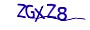×

# PHP 面向对象 final类与final方法 不允许继承

#####chen发表于2021-07-30 12:00:32浏览79评论0

final---用于类、方法前。
final类---不可被继承。
final方法---不可被覆盖。
final类不能被继承。

<?
//声明一个final类Math
final class Math{
public static \$pi = 3.14;

public function __toString(){
return "这是Math类。";
}
}
\$math = new Math();
echo \$math;

//声明类SuperMath 继承自 Math类
class SuperMath extends Math {
}
//执行会出错，final类不能被继承。

?>

Fatal error: Class SuperMath may not inherit from final class (Math) in

final方法不能被重写

<?
//声明一个final类Math
class Math{
public static \$pi = 3.14;
public function __toString(){
return "这是Math类。";
}
public final function max(\$a,\$b){
return \$a > \$b ? \$a : \$b ;
}
}
//声明类SuperMath 继承自 Math类
class SuperMath extends Math {
public final function max(\$a,\$b){}
}
//执行会出错，final方法不能被重写。

?>

Fatal error: Class SuperMath may not inherit from final class (Math) in

### 群贤毕至